# Chemistry Assignment Help with Measurement of Entropy

## 9.16 Measurement of Entropy and Enthalpy Changes from EMF Data

From Gibbs-Helmholtz equation we have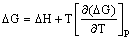DG = – nEF and differentiating DG with respect to temperature at constant pressure yields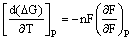The quantity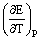is the temperature coefficient of the cell. Substituting the value of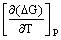in equation, we get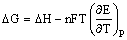Also DG = DH – TDS

Hence from equations, we obtain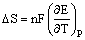Equation can be used for calculating the entropy changes for the cell reaction in terms of the temperature coefficient of the cell emf. Equation can also be written as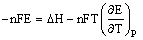or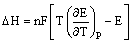From equation, the enthalpy change for cell reaction can be determined from the measurement of cell emf and the temperature coefficient of the emf.### Email Based Assignment Help in Measurement of Entropy

We are the leading online Assignment Help provider. Find answers to all of your doubts regarding the Measurement of Entropy in chemistry. We at assignmenthelp.net provide homework, Assignment Help to the school, college or university level students. Our expert online tutors are available to help you in Measurement of Entropy. Our service is focused on: time delivery, superior quality, creativity and originality.